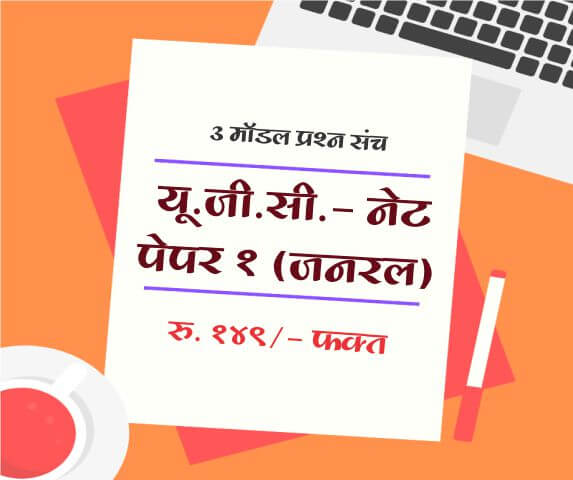Calculator
 Calculate Speed of Train (S) Speed of Object (SO) Time Taken (T) Length of Train (L) Length of Object (W)
 Object Direction Same DirectionOpposite Direction Object Speed (SO) in KM/hr Time Taken (T) in Seconds Train Length (L) Length of Object (0 if Human)

Train passing Moving object

Train having length of L including engine when passes a moving object the time taken by train to pass moving object with length W is calculated as follows

If train and object going parallel in same direction then formula will be as follows
Time taken by train to pass object is T= (Length of Train L + length of moving object W) / ( Speed of train S - Speed of Object SO)
i.e. T = ( L + W ) / (S - SO)

If train and object going parallel in opposite direction then formula will be as follows
Time taken by train to pass object is T= (Length of Train L + length of moving object W) / ( Speed of train S + Speed of Object SO)
i.e. T = ( L + W ) / (S + SO)

The moving objects can be a train, car, man.

 Name : Comment :

Calculators

Subscribe to get latest updates about Exam Schedules, Government Jobs, General Knowledge & Current Affairs and Many more on Whatsapp!Full Length Mock Tests
Timer Based Exams
Instant Result and assesment
Detailed analasys of ResultUGC NET SET Paper 1 Mock Tests

UGC-NET / SET Paper 1 mock test

3 Mock Tests, 150 Questions with Explanations

Rs: 149 Only/-LAW MH-CET 2019 (3 YEAR COURSE)

Mock test for MH CET Law 2019 for three year law programme

3 Mock Tests, 450 Questions with Explanations

Rs: 249 Only/-यू. जी. सी. - नेट पेपर १ (जनरल)

यू. जी. सी. - नेट पेपर १ (अनिवार्य) सामान्य प्रश्न हिंदीमें

3 Mock Tests, 150 Questions with Explanations

Rs: 149 Only/-